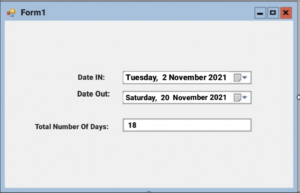# Find difference between Two dates in C#

Create a simple windows application that will take two dates as input and display the difference between two dates in C#.

### Difference Between Two Dates in C# WinForms

If we want to find how many days are in between any two of the given dates or simply we can say the difference between the two dates it can be calculate with some methods that are predefined in many of the languages.

In C# calling any of the overloads of the DateTime constructor will allow you to specify specific elements of the date and time value like year , month or day. DateTime newDate = new DateTime(2000, 8, 1);

Here new DateTime represents year is 2000 and month (5) means August and date is 1.

#### DateTime structure/method:

Using a DateTime structure for Calculation, like Add or Subtract, does not modify the value of the structure but it returns a new DateTime structure whose value is the result of the calculation.

The method is used to returns a new DateTime that adds the value of the specified TimeSpan to the value of this instance.
• DateTime.Subtract():
The method is used in order to find the date-time difference between two instances of the DateTime method.
`Dim diff As System.TimeSpan = secondDate.Subtract(firstDate)`
##### You can also find the difference between two dates by using (-) operator:
`Dim diff2 As String = (secondDate - firstDate).TotalDays.ToString()`

In above example it is using a subtraction operator (-) for doing the operation.

#### Convert String to DateTime

We can use the methods like Convert.ToDateTime(String), DateTime.Parse() and DateTime.ParseExact() methods for converting a string-based date into a System.DateTime object.

## C# program to find difference between two dates

```using System;
using System.Windows.Forms;

namespace WindowsFormsApplication1
{
public partial class Form1 : Form
{
public Form1()
{
InitializeComponent();
dateTimePicker1.ValueChanged +=new EventHandler(dateTimePicker1_ValueChanged);
dateTimePicker2.ValueChanged += new EventHandler(dateTimePicker1_ValueChanged);
}
private void dateTimePicker1_ValueChanged(object sender, EventArgs e)
{
DateTime inTime = Convert.ToDateTime(dateTimePicker1.Text);
DateTime outTime = Convert.ToDateTime(dateTimePicker2.Text);
if (outTime >= inTime)
{
textBox1.Text = outTime.Subtract(inTime).Days.ToString();
}
}
}
}```

On the form1, take three lables and name them Date IN, Date Out and Total Number of Days , and two DateTime picker control which will take the inputs from user in date time format and after calculation it will display the result in the text box.

##### Output screen:Now run the application and when we choose two dates it displays the answer in the text box.

As you can see in the above example I have pick two dates which are 2 November 2021 and 20 November 2021 and it’s showing the result 18 in the total no. of days text box.

Subtraction method calculate the difference between two dates. Everytime result will be in days, but it can be zero if the both dates are same.

So in this way we can simply find the difference between the two dates in C# Windows Application.